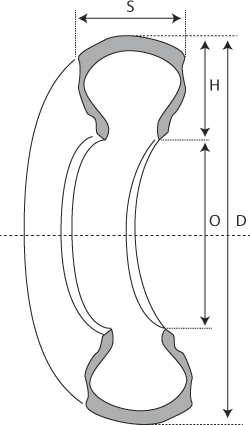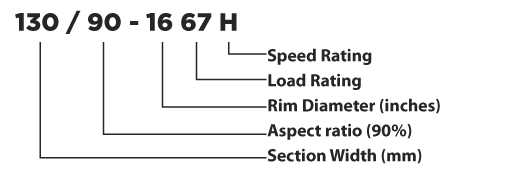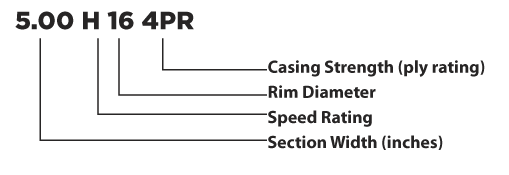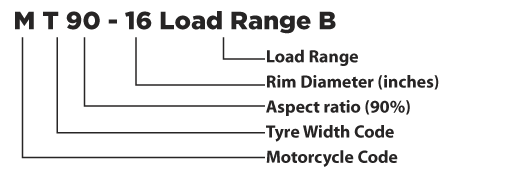## WHAT TYRE DESIGNATIONS MEAN

Aspect Ratio

The aspect ratio of a tyre is the relationship of the tyre cross section height (H) to the cross section width (S).

In a tyre with an aspect ratio of 85, height is about 85% of the tyre width. 'Low profile' tyres have an aspect ratio of less than 80. The aspect ratio of a tyre is often contained in the size marking, e.g. 130/90 x 16.Aspect Ratio = ( H / S) x 100

D = Overall diameter
H= Section Height of the tyre
S = Max section width of the tyre
O = Rim diameter at the bead seam

Street Tyre Size Designations

METRIC:INCH:ALPHABETICAL:Speed Rating (Sustained)

R = 106mph
S = 112mph
T = 118mph
H = 130mph
V = 150mph
Z = 150+ mph